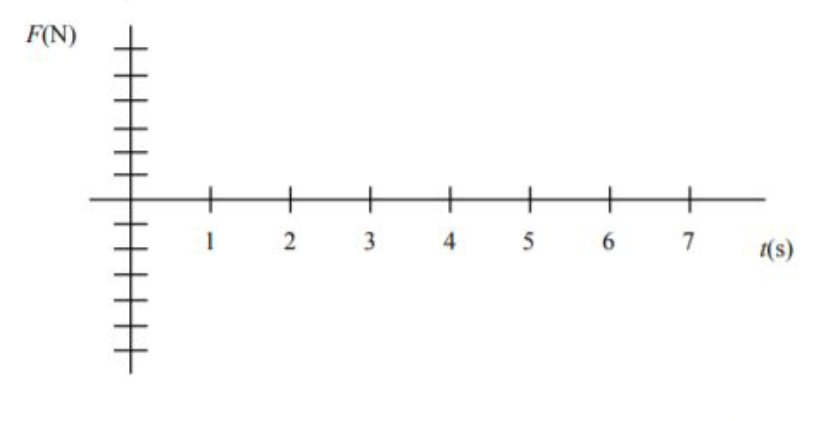# Oscillations#

A simple harmonic oscillator has a displacement from equilibrium $$x = A \cos(\phi)$$, where the phase is plotted as a function of time in Fig. 1.## Part 1#

What is the frequency of this oscillation?

• 10 $$s$$

• 0.10 $$Hz$$

• 0.1 $$\pi$$ $$Hz$$

• 0.05 $$Hz$$

• 20 $$s$$